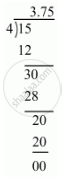Share

# Express the Following Rational Number as Decimal: 15/4 - CBSE Class 9 - Mathematics

ConceptIntroduction of Real Number

#### Question

Express the following rational number as decimal:

15/4

#### Solution

Given rational number is 15/4

Now we have to express this rational number into decimal form. So we will use long division method as below.Hence, 15/4=3.75

Is there an error in this question or solution?

#### APPEARS IN

Solution Express the Following Rational Number as Decimal: 15/4 Concept: Introduction of Real Number.
S